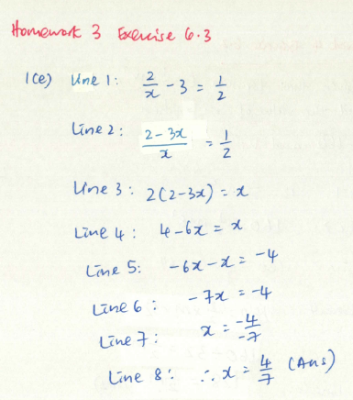### Chap 12 (ICT Investigation 1) Introduction to Linear Graphs

In pairs, you are going to investigate the change of the "c" in the linear equation "y = mx + c" to find out what it is.

Instructions:
1. Login to GoogleSite (Mathematics > Class Page) to download the file (Chap 12 ICT Investigation 1.ggb).

2. Open the file, you will see 3 lines.
• The 2 black lines represent equations y = 2x + 5 and y = 2x -2 while the pink line can "move".
• The equations of the line are displayed on the left panel.

3. Drag the slider (on top) to change the value of n (for the pink line) to 3, 2, 1, 0, -1, -2.

Discuss your observation on:
1. Describe the 'relationship' between the pink line and the 2 other lines?
2. Does the orientation of the line change as we drag the slider?
3. What happens to the equation of the pink line as you drag the slider to the different numbers?
4. Make a link between the position of the pink line and the equation.
Submit the observation under "Comments".
Remember to sign off with your name and your partner(s)'s name before submitting your observation.

### Chap 12 (ICT Investigation 2) Introduction to Linear Graphs

In pairs, you are going to investigate the change of the "m" in the linear equation "y = mx + c" to find out what it is.

Instructions:
1. Login to GoogleSite (Mathematics > Class Page) to download the file (Chap 12 ICT Investigation 2.ggb).
2. Open the file, you will see 2 lines.
• The black line that represents the equation y = x is fixed (it will not move) whereas the move line moves as you drag the slider.
• The equations of the line are displayed on the left panel.3. Drag the slider (on top) to change the value of n (for the pink line) to 3, 2, 1, 0, -1, -2.

Discuss your observation on:
1. Describe the 'relationship' between the pink line and the 2 other lines?
2. Does the orientation of the line change as we drag the slider?
3. What happens to the equation of the blue line as you drag the slider to the different numbers?
4. Make a link between the position of the blue line and the equation.
Submit the observation under "Comments".
Remember to sign off with your name and your partner(s)'s name before submitting your observation.

### Chap 12 (ICT Investigation) Study Notes (p9-p11)

Variation in the value of m with the value of c kept constant

Increase the value of m. What do you observe about the straight line?
As m increases, the slope becomes steeper.

Now decrease the value of m. What do you observe about the straight line?
As m decreases, the slope becomes more gentle.

Now further decrease the value of m till it comes negative. What do you observe about the straight line?
The line continues to "go down": Now the axis points from top left corner towards bottom right corner. (opposite direction).

Now set the value m = 0. What do you observe about the straight line?
When m becomes zero, the line becomes the horizontal line that overlaps with the x axis.

Variation in the value of c with the value of m kept constant

Increase the value of c. What do you observe about the straight line?
The straight line 'moves' up, and it remains parallel to the original line.

Now decrease the value of c. What do you observe about the straight line?
The straight line 'moves' up, and it remains parallel to the original line.

What happens to the straight line when the value of c = 0?
The straight one passes through the origin.

Now summarize on your own the effect of m and c on a straight line with equation y = mx + c

c is the vertical intercept that tells us where the graph will intersect/ cut the y axis whereas m is the gradient that tells us the orientation of the graph (steep/ gentle, pointing upwards/ downwards).

What happen if now we have two lines having the same gradient?
Consider y = 2x + 4 and y = 2x - 1

What happen if now we have two lines having the same y-intercept?
Consider y = 2x + 1 and y = -4x + 1The two line will cut the y-axis at y = 1

What is the general form of a horizontal straight line?
Why is it in that particular form?
The general form is y = c when c is the 'y'-intercept
i.e. when the line is parallel to the x axis.

What is the general form of a vertical straight line?
What is it in that particular form?
The general form is x = n where n is the 'x'-intercept
i.e. when the line is parallel to the y axis.

### Chap 12: Plotting of Graphs (Study Notes p12 Example 12.4A)

Plotting of Linear Graphs

The following components are essential (p11)
• Table of 3 points (3-point plot)
• Determine the "domain" and "range": the maximum and minimum values of the x (horizontal) and y (vertical) axis
• Scale of the graph
• Plotting the points and drawing a straight line that passes through the points
A good scale of the graph is when the domain and range covers 2/3 to 3/4 of the graph paper.

### Chap 12: Plotting of Graphs (Study Notes p12 Practice 3)

On a piece of graph paper, plot the line y = 4x + 2 where -2 ≤ x ≤ 5

Your graph should look like this: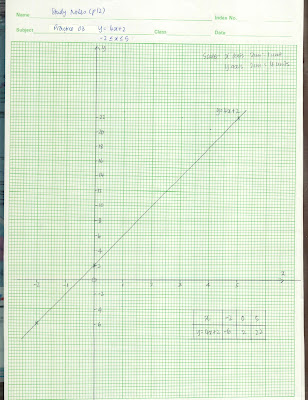### Chap 16 (Activity 1) What does the line say?With reference to the graph above, give a scenario that could be represented by the graph above.
Remember to 'name' the horizontal and vertical axes so that you could describe the relationship clearly.
At the end of the description, make an attempt to write down the equation for the graph (with y being the vertical axis, x being the horizontal axis)

The relationship between the horizontal (x) and vertical (y) axes: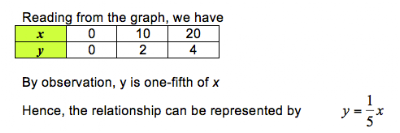### Chap 16 (Activity 2) What does the line say?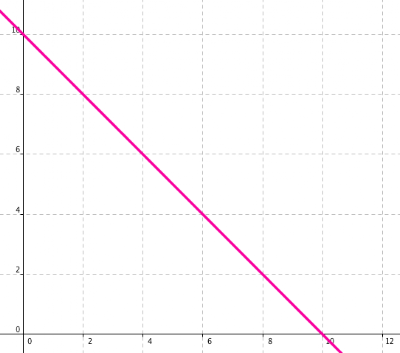With reference to the graph above, give a scenario that could be represented by the graph above.
Remember to 'name' the horizontal and vertical axes so that you could describe the relationship clearly.
At the end of the description, make an attempt to write down the equation for the graph (with y being the vertical axis, x being the horizontal axis)

The relationship between the horizontal (x) and vertical (y) axes: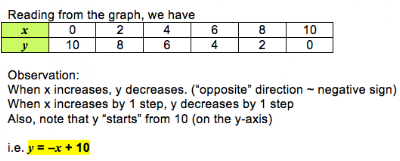### Chap 12: Graph Mole

Read the "Introduction" to find out the context and the "how-to" of the game before "playing the game" to challenge yourself!
It's a "combination" of knowledge and speed! Enjoy!
Link: http://funbasedlearning.com/algebra/graphing/points/### Chap 12: What's the Point?

Let's try this... of course, pick the most challenging one to practise!

Link: FunBrain.com's What's the Point?### Chap 12: Coordinates And Linear Graphs - Can you find the Dinosaurs?

Your Mission
Applying what you know, be the first to find all 7 "dinos".
Reporting your findings... {under comments}
e.g. Dino #1: (.... , ....), (.... , ....), (.... , ....)
e.g. Dino #2: (.... , ....), (.... , ....), (.... , ....)
e.g. Dino #3: (.... , ....), (.... , ....), (.... , ....)
e.g. Dino #4: (.... , ....), (.... , ....), (.... , ....)
e.g. Dino #5: (.... , ....), (.... , ....), (.... , ....)
e.g. Dino #6: (.... , ....), (.... , ....), (.... , ....)
e.g. Dino #7: (.... , ....), (.... , ....), (.... , ....)

Note:
You may have less than or more than 3 ordered pairs to report the location of each dino you found.

### 20120717 Homework

(1) Post your thoughts to the Question: Chap 6 Anything Wrong?

(2) Error Analysis - Spot the errors to figure out what's wrong with the working.

(3) Challenging Practice (From Maths Workbook (p35)
Choose ONE question to answer (Q21 to Q25)
Work out the answers (with steps clearly shown) on paper.
Take a picture of your answers and post it in the Maths blog

Subject Title: Chap 6 Simple Equations Challenging Practice Question #26 {enter the question number that you are doing} by MY_NAME
Label: algebra, equation

Complete the homework by today (2012, July 17).
(Remember: Do not accumulate your homework because you need time to do your revision)

### Support Programme on 17 July 2012

Dear students

To facilitate the taking place of the GCE "O" Level Examination for Mother Tongue Language Listening Comprehension, there will be NO Face-to-Face Maths Support Programme tomorrow.

Nevertheless, printed handouts (Algebra IV) will be given to you for practice (to prepare for the Level Test). It will be given to you tomorrow.

Please complete the worksheet and check the answers (available at GoogleSite > Mathematics > Support Programme (Sec 1) [at the left panel]

I will be available on Thurdsay from 2 pm to 3 pm at the Info Hub. You may see me at there to clarify your doubts. Alternatively, you may 'look' for me through "Facebook" or "Email".

Cheers! Ms Loh

### Chap 6 Homework Submission

Topic: Algebra - Simple Linear Equations in one unknown

The following have not submitted Homework 1-4

Homework 1: Ex 6.1
1. A Manicka Praveen
2. Chiam Chuen

Homework 2: Ex 6.2
1. A Manicka Praveen
2. Chiam Chuen

Homework 3: Ex 6.3
1. Chiam Chuen
2. Goh Qian Zhe
3. Khor Ethan
4. Ng Keen Yung

Homework 4: Ex 6.2 & Ex 6.3
1. Chiam Chuen
2. Ng Keen Yung

ICT Investigation
1. A Manicka Praveen
2. Koh Guo Feng

### Chap 6: Anything Wrong?

Antonio, Benedict and Celine were tasked to factorise the following:
40ax - 10bx - 60ay + 15by
and all three presented different answers.

Antonio. (10x - 15y)(4a - b)
Benedict. (2x - 3y)(20a - 5b)
Celine. 5(3y - 2x)(b - 4a)

Discuss the solution by each student and determine if each is correct.
- if the answer is correct, describe how he/ she arrived at that answer
- if the answer is incomplete, how should he/she continue from there
- if the answer is incorrect, point out what is the likely mistake

### Chap 6 Solving Simple Linear Equation : Working that is not appropriate

Below is a sample of working that does not show that we are solving linear equation.
It's just arithmetic operation and does not explain the train of thoughts clearly how we solve the solution.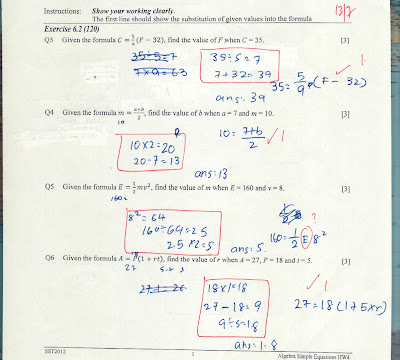### Chap 6 Error Analysis Homework 2 Ex 6.2 Q1(f)

Go through the working and attempt to identify where the error is.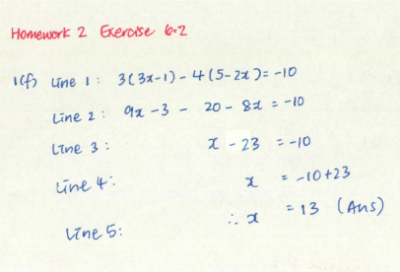### Chap 6 Error Analysis Homework 2 Ex 6.2 Q1(j)

Go through the working and attempt to identify where the error is.### Chap 6 Error Analysis Homework 2 Ex 6.2 Q1(l) #1

Go through the working and attempt to identify where the error is.### Chap 6 Error Analysis Homework 2 Ex 6.2 Q1(l) #2

Go through the working and attempt to identify where the error is.### Chap 6 Error Analysis Homework 2 Ex 6.2 Q2(a) #1

Go through the working and attempt to identify where the error is.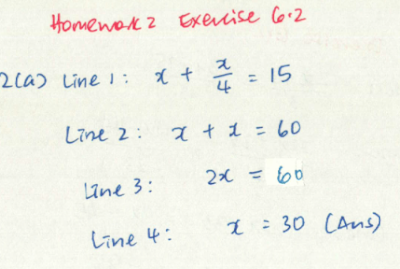### Chap 6 Error Analysis Homework 2 Ex 6.2 Q2(a) #2

Go through the working and attempt to identify where the error is.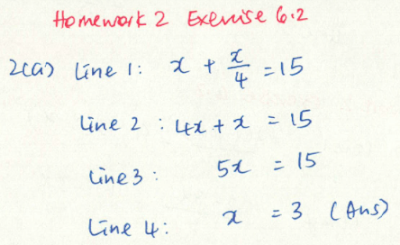### Chap 6 Error Analysis Homework 2 Ex 6.2 Q2(c)

Go through the working and attempt to identify where the error is.### Chap 6 Error Analysis Homework 2 Ex 6.2 Q2(e)

Go through the working and attempt to identify where the error is.### chap 6 Error Analysis Homework 2 Ex 6.3 Q1(e) #1

Go through the working and attempt to identify where the error is.### chap 6 Error Analysis Homework 2 Ex 6.3 Q1(e) #2

Go through the working and attempt to identify where the error is.